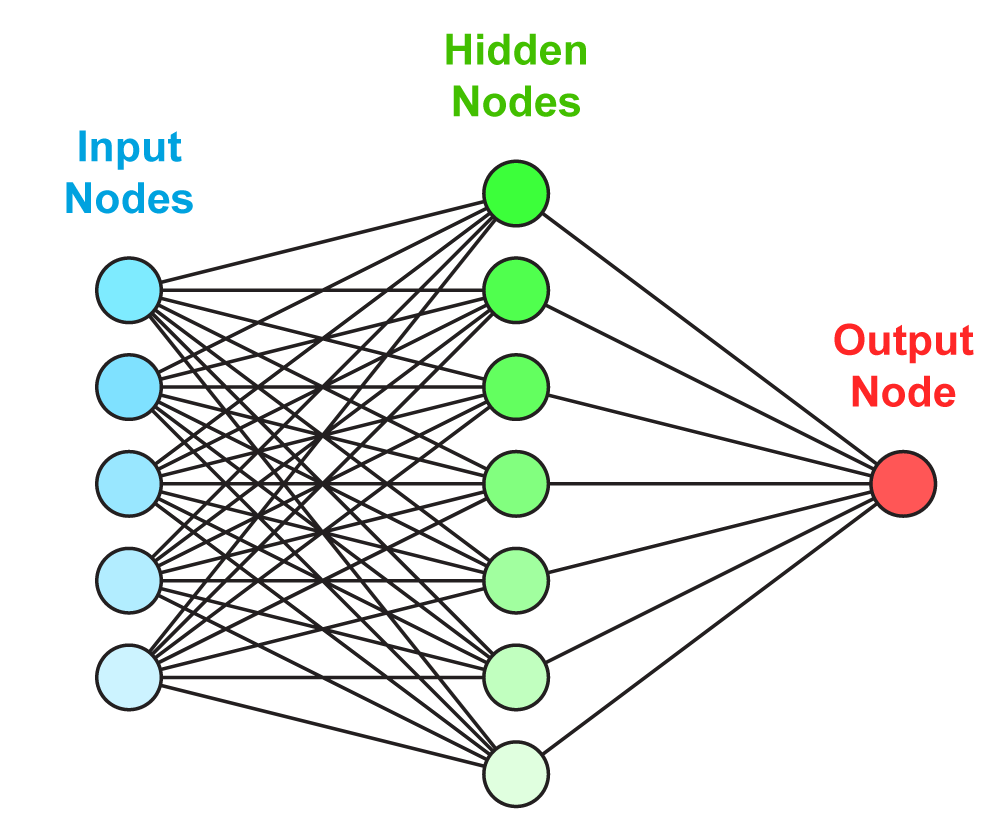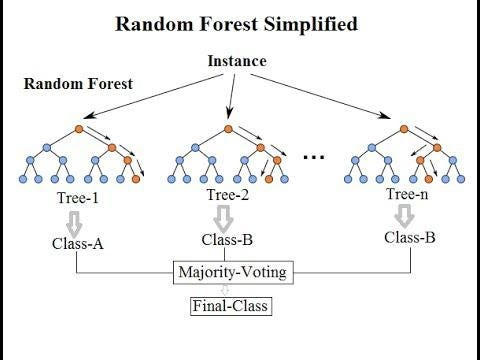Deep Learning

# 3 Reasons to Use Random Forest Over a Neural Network: Comparing Machine Learning versus Deep Learning

March 3, 2020Neural networks have been shown to outperform a number of machine learning algorithms in many industry domains. They keep learning until it comes out with the best set of features to obtain a satisfying predictive performance. However, a neural network will scale your variables into a series of numbers that once the neural network finishes the learning stage, the features become indistinguishable to us.If all we cared about was the prediction, a neural net would be the de-facto algorithm used all the time. But in an industry setting, we need a model that can give meaning to a feature/variable to stakeholders. And these stakeholders will likely be anyone other than someone with a knowledge of deep learning or machine learning.

#### What’s the Main Difference Between Random Forest and Neural Networks?

Both the Random Forest and Neural Networks are different techniques that learn differently but can be used in similar domains. Random Forest is a technique of Machine Learning while Neural Networks are exclusive to Deep Learning.

#### What are Neural Networks?

A Neural Network is a computational model loosely based on the functioning cerebral cortex of a human to replicate the same style of thinking and perception. Neural Networks are organized in layers made up of interconnected nodes that contain an activation function that computes the output of the network.Neural nets are another means of machine learning in which a computer learns to perform a task by analyzing training examples. As the neural net is loosely based on the human brain, it will consist of thousands or millions of nodes that are interconnected. A node can be connected to several nodes in the layer beneath it, from which it receives data and several nodes above it which receive data. Each incoming data point receives a weight and is multiplied and added. A bias is added if the weighted sum equates to zero and then passed to the activation function.#### The Architecture of Neural Networks

A Neural Network has 3 basic architectures:

1. Single Layer Feedforward Networks
• It is the simplest network that is an extended version of the perceptron. It has additional hidden nodes between the input layer and the output layer.

2. Multi-Layer Feedforward Networks

• This type of network has one or more hidden layers except for the input and output. Its role is to intervene in data transfer between the input and output layers.

3. Recurrent Networks

• Recurrent neural networks are similar to the above but are widely adopted to predict sequential data such as text and time series. The most famous Recurrent Neural Network is the ‘Long — Short Term Memory’ Model (LSTM).

#### What is Random Forest?Random Forest is an ensemble of Decision Trees whereby the final/leaf node will be either the majority class for classification problems or the average for regression problems.

A random forest will grow many Classification trees and for each output from that tree, we say the tree ‘votes’ for that class. A tree is grown using the following steps:

1. A random sample of rows from the training data will be taken for each tree.
2. From the sample taken in Step (1), a subset of features will be taken to be used for splitting on each tree.
3. Each tree is grown to the largest extent specified by the parameters until it reaches a vote for the class.

#### Why Should You Use Random Forest?

The fundamental reason to use a random forest instead of a decision tree is to combine the predictions of many decision trees into a single model. The logic is that a single even made up of many mediocre models will still be better than one good model. There is truth to this given the mainstream performance of random forests. Random forests are less prone to overfitting because of this.

Over-fitting can occur with a flexible model like decision trees where the model with memorizing the training data and learn any noise in the data as well. This will make it unable to predict the test data.

A random forest can reduce the high variance from a flexible model like a decision tree by combining many trees into one ensemble model.

#### When Should You Use Random Forest Versus a Neural Network?

Random Forest is less computationally expensive and does not require a GPU to finish training. A random forest can give you a different interpretation of a decision tree but with better performance. Neural Networks will require much more data than an everyday person might have on hand to actually be effective. The neural network will simply decimate the interpretability of your features to the point where it becomes meaningless for the sake of performance. While that may sound reasonable to some, it is dependent on each project.

If the goal is to create a prediction model without care for the variables at play, by all means, use a neural network, but you’ll need the resources to do so. If an understanding of the variables is required, then whether we like it or not, typically what happens in this situation is that performance will have to take a slight hit to make sure that we can still understand how each variable is contributing to the prediction model.### Free Resources

Browse our whitepapers, e-books, case studies, and reference architecture.

#### Have any questions?Deep Learning

## 3 Reasons to Use Random Forest Over a Neural Network: Comparing Machine Learning versus Deep Learning

Neural networks have been shown to outperform a number of machine learning algorithms in many industry domains. They keep learning until it comes out with the best set of features to obtain a satisfying predictive performance. However, a neural network will scale your variables into a series of numbers that once the neural network finishes the learning stage, the features become indistinguishable to us.If all we cared about was the prediction, a neural net would be the de-facto algorithm used all the time. But in an industry setting, we need a model that can give meaning to a feature/variable to stakeholders. And these stakeholders will likely be anyone other than someone with a knowledge of deep learning or machine learning.

#### What’s the Main Difference Between Random Forest and Neural Networks?

Both the Random Forest and Neural Networks are different techniques that learn differently but can be used in similar domains. Random Forest is a technique of Machine Learning while Neural Networks are exclusive to Deep Learning.

#### What are Neural Networks?

A Neural Network is a computational model loosely based on the functioning cerebral cortex of a human to replicate the same style of thinking and perception. Neural Networks are organized in layers made up of interconnected nodes that contain an activation function that computes the output of the network.Neural nets are another means of machine learning in which a computer learns to perform a task by analyzing training examples. As the neural net is loosely based on the human brain, it will consist of thousands or millions of nodes that are interconnected. A node can be connected to several nodes in the layer beneath it, from which it receives data and several nodes above it which receive data. Each incoming data point receives a weight and is multiplied and added. A bias is added if the weighted sum equates to zero and then passed to the activation function.#### The Architecture of Neural Networks

A Neural Network has 3 basic architectures:

1. Single Layer Feedforward Networks
• It is the simplest network that is an extended version of the perceptron. It has additional hidden nodes between the input layer and the output layer.

2. Multi-Layer Feedforward Networks

• This type of network has one or more hidden layers except for the input and output. Its role is to intervene in data transfer between the input and output layers.

3. Recurrent Networks

• Recurrent neural networks are similar to the above but are widely adopted to predict sequential data such as text and time series. The most famous Recurrent Neural Network is the ‘Long — Short Term Memory’ Model (LSTM).

#### What is Random Forest?Random Forest is an ensemble of Decision Trees whereby the final/leaf node will be either the majority class for classification problems or the average for regression problems.

A random forest will grow many Classification trees and for each output from that tree, we say the tree ‘votes’ for that class. A tree is grown using the following steps:

1. A random sample of rows from the training data will be taken for each tree.
2. From the sample taken in Step (1), a subset of features will be taken to be used for splitting on each tree.
3. Each tree is grown to the largest extent specified by the parameters until it reaches a vote for the class.

#### Why Should You Use Random Forest?

The fundamental reason to use a random forest instead of a decision tree is to combine the predictions of many decision trees into a single model. The logic is that a single even made up of many mediocre models will still be better than one good model. There is truth to this given the mainstream performance of random forests. Random forests are less prone to overfitting because of this.

Over-fitting can occur with a flexible model like decision trees where the model with memorizing the training data and learn any noise in the data as well. This will make it unable to predict the test data.

A random forest can reduce the high variance from a flexible model like a decision tree by combining many trees into one ensemble model.

#### When Should You Use Random Forest Versus a Neural Network?

Random Forest is less computationally expensive and does not require a GPU to finish training. A random forest can give you a different interpretation of a decision tree but with better performance. Neural Networks will require much more data than an everyday person might have on hand to actually be effective. The neural network will simply decimate the interpretability of your features to the point where it becomes meaningless for the sake of performance. While that may sound reasonable to some, it is dependent on each project.

If the goal is to create a prediction model without care for the variables at play, by all means, use a neural network, but you’ll need the resources to do so. If an understanding of the variables is required, then whether we like it or not, typically what happens in this situation is that performance will have to take a slight hit to make sure that we can still understand how each variable is contributing to the prediction model.### Free Resources

Browse our whitepapers, e-books, case studies, and reference architecture.